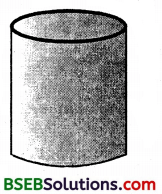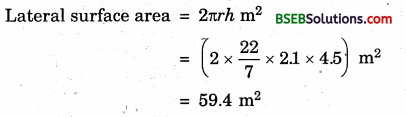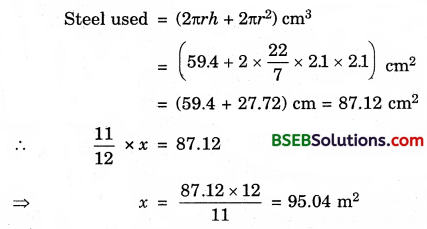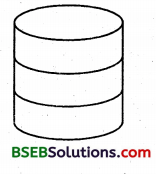# HSSlive: Plus One & Plus Two Notes & Solutions for Kerala State Board

## BSEB Class 9 Maths Chapter 13 Surface Areas and Volumes Ex 13.2 Textbook Solutions PDF: Download Bihar Board STD 9th Maths Chapter 13 Surface Areas and Volumes Ex 13.2 Book AnswersBSEB Class 9 Maths Chapter 13 Surface Areas and Volumes Ex 13.2 Textbook Solutions PDF: Download Bihar Board STD 9th Maths Chapter 13 Surface Areas and Volumes Ex 13.2 Book Answers

BSEB Class 9th Maths Chapter 13 Surface Areas and Volumes Ex 13.2 Textbooks Solutions and answers for students are now available in pdf format. Bihar Board Class 9th Maths Chapter 13 Surface Areas and Volumes Ex 13.2 Book answers and solutions are one of the most important study materials for any student. The Bihar Board Class 9th Maths Chapter 13 Surface Areas and Volumes Ex 13.2 books are published by the Bihar Board Publishers. These Bihar Board Class 9th Maths Chapter 13 Surface Areas and Volumes Ex 13.2 textbooks are prepared by a group of expert faculty members. Students can download these BSEB STD 9th Maths Chapter 13 Surface Areas and Volumes Ex 13.2 book solutions pdf online from this page.

## Bihar Board Class 9th Maths Chapter 13 Surface Areas and Volumes Ex 13.2 Books Solutions

 Board BSEB Materials Textbook Solutions/Guide Format DOC/PDF Class 9th Subject Maths Chapter 13 Surface Areas and Volumes Ex 13.2 Chapters All Provider Hsslive

## How to download Bihar Board Class 9th Maths Chapter 13 Surface Areas and Volumes Ex 13.2 Textbook Solutions Answers PDF Online?

2. Click on the Bihar Board Class 9th Maths Chapter 13 Surface Areas and Volumes Ex 13.2 Answers.
3. Look for your Bihar Board STD 9th Maths Chapter 13 Surface Areas and Volumes Ex 13.2 Textbooks PDF.
4. Now download or read the Bihar Board Class 9th Maths Chapter 13 Surface Areas and Volumes Ex 13.2 Textbook Solutions for PDF Free.

## BSEB Class 9th Maths Chapter 13 Surface Areas and Volumes Ex 13.2 Textbooks Solutions with Answer PDF Download

Find below the list of all BSEB Class 9th Maths Chapter 13 Surface Areas and Volumes Ex 13.2 Textbook Solutions for PDF’s for you to download and prepare for the upcoming exams:

## BSEB Bihar Board Class 9th Maths Solutions Chapter 13 Surface Areas and Volumes Ex 13.2

Question 1.
The curved surface area of a right circular cylinder of height 14 cm is 88 cm². Find the diameter of the base of the cylinder.
Solution:
Let r be the radius of the base and h = 14 cm be the height of the cylinder. Then,
Curved surface area = 2πrhQuestion 2.
It is required to make a closed cylindrical tank of height 1 m and base diameter 140 cm from a metal sheet. How many square metres of the sheet are required for the same?
Solution:
Let r be the radius of the base and h be the height of the cylinder.
Metal sheet required to make a closed cylindrical tank = Its total surface areaHence, the sheet required = 7.48 m².

Question 3.
A metal pipe is 77 cm long. The inner diameter of a cross-section is 4 cm, the outer diameter being 4.4 cm (see figure). Find its(i) inner curved surface area,
(ii) outer curved surface area,
(iii) total surface area.
Solution:
We have, R = external radius = 4.42 cm = 2.2 cm
r = internal radius = 42 cm = 2 cm
h = length of the pipe = 77 cm.
(i) Inner curved surface = 2πrh cm²
= 2 x 227 x 2 x 77 cm²
= 968 cm²

(ii) Outer curved surface = 2πRh cm²
= 2 x 227 x 2.2 x 77 cm²
= 1064.8 cm²

(iii) Total surface area of a pipe = Inner curved surface area + outer curved surface area + areas of two basesQuestion 4.
The diameter of a roller is 84 cm and its length is 120 cm. It takes 500 complete revolutions to move once over to level a playground. Find the area of the playground in m².
Solution:
The length of the roller is 120 cm i.e., h = 1.2 m and radius of the cylinder (i.e., roller) = 842 cm = 42 cm = 0.42 m.
Distance covered by roller in one revolution
= Its curved surface area
= 2πrh
= (2 x 227 x 0.42 x 1.2) m²
= 3.168 m²
Area of the playground = Distance covered by roller in 500 revolution
= (500 x 3.168) m²
= 1584 m²

Question 5.
A cylindrical pillar is 50 cm in diameter and 3.5 m in height. Find the cost of painting the curved surface of the pillar at the rate of Rs 12.50 per m².
Solution:
Let r be the radius of the base and h be the height of the pillar.
∴ r = 502 cm = 25 cm = 25 m and h = 3.5 m.
Curved surface = 2πrh
= (2 x 227 x 0.25 x 3.5) m²
= 5.5 m²
Cost of painting the curved surface @ Rs 12.50 per m²

Question 6.
Curved surface area of a right circular cylinder is 4.4 m². If the radius of the base of the cylinder is 0. 7 m, find its height.
Solution:
Let r be the radius of the base and h be| the height of the cylinder. Then,
Curved surface area = 4.4 m²
⇒ 2πrh = 4.4
⇒ 2 x 227 x 0.7 x h = 4.4 [∵ r = 0.7 m]
⇒ h = (4.4×72×22×0.7) m = 1 m
Thus, the height of the cylinder = 1 metre.

Question 7.
The inner diameter of a circular well is 3.5 m. It is 10 m deep. Find
(i) its inner curved surface area,
(ii) the cost of plastering this curved surface at the rate of Rs 40 per m².
Solution:
(i) Let r be the radius of the face and h be depth of the well. Then,
Curved surface = 2nrh
= (2 x 227 x 3.52 x 10) m² = 110 m²

(ii) Cost of plastering is Rs 40 per m²
∴Cost of plastering the curved surface = Rs (110 x 40)
= Rs 4400.

Question 8.
In a hot water heating system, there is a cylindrical pipe Pf length 28 m and diameter 5 cm. Find the total radiating surface in the system.
Solution:
Total radiating surface in the system
= Curved surface area of the pipe
= 2πrh,
where r = 52 cm = 2.5 cm = 2.5100 m = 0.025 m and h = 28 m
= (2 x 227 x 0.025 x 28) m² = 4.4 m²

Question 9.
Find (i) the lateral or curved surface.area of a cylindrical petrol storage tank that is 4.2 m in diameter and 4.5 m high.
(ii) how much steel was actually used, if 112 of the steel actually used was wasted in making the closed tank.
Solution:
(i) Here, r = (4.22)m = 2.1 m and h = 4.5 m.(ii) Since 112 of the actual steel used was wasted, the area of the steel which has gone into the tank = (1 – 112) of x = 1112 of x.Hence, the actual area of the steel used = 95.04 m²

Question 10.
In figure, you see the frame of a lampshade. It is to be covered with a decorative cloth. The frame has a base diameter of 20 cm and height of 30 cm. A margin of 2.5 cm is to be given for folding it over the top and bottom of the frame. Find how much cloth is required for covering the lampshade.Solution:
Here r = (202) cm = 10 cm and h = 30 cm + 2 x 2.5 cm (i.e margin) = 35 cm.
Cloth required for covering the lampshade
= Its curved surface area
= 2πrh
= (2 x 227 x 10 x 35) cm²
= 2200 cm²

Question 11.
The students of a Vidyalaya were asked to participate in a competition for making and decorating penholders in the shape of a cylinder with a base, using cardboard. Each penholder was to be of radius 3 cm and height 10.5 cm. The Vidyalaya was to supply the competitors with cardboard. If there were 35 competitors, how much cardboard was required to be bought for the competition?
Solution:
Cardboard required by each competitor = Curved surface area of one penholder + base area
= 2πrh + πr² where r = 3 cm, h = 10.5 cm
= [(2 x 227 x 3 x 10.5) + 227 x 9] cm²
= (198 + 28.28) cm²
= 226.28 cm² (approx.)
Cardboard required for 35 competitors = (35 x 226.28) cm²
= 7920 cm² (approx.)

## Bihar Board Class 9th Maths Chapter 13 Surface Areas and Volumes Ex 13.2 Textbooks for Exam Preparations

Bihar Board Class 9th Maths Chapter 13 Surface Areas and Volumes Ex 13.2 Textbook Solutions can be of great help in your Bihar Board Class 9th Maths Chapter 13 Surface Areas and Volumes Ex 13.2 exam preparation. The BSEB STD 9th Maths Chapter 13 Surface Areas and Volumes Ex 13.2 Textbooks study material, used with the English medium textbooks, can help you complete the entire Class 9th Maths Chapter 13 Surface Areas and Volumes Ex 13.2 Books State Board syllabus with maximum efficiency.

## FAQs Regarding Bihar Board Class 9th Maths Chapter 13 Surface Areas and Volumes Ex 13.2 Textbook Solutions

#### How to get BSEB Class 9th Maths Chapter 13 Surface Areas and Volumes Ex 13.2 Textbook Answers??

Students can download the Bihar Board Class 9 Maths Chapter 13 Surface Areas and Volumes Ex 13.2 Answers PDF from the links provided above.

#### Can we get a Bihar Board Book PDF for all Classes?

Yes you can get Bihar Board Text Book PDF for all classes using the links provided in the above article.

## Important Terms

Bihar Board Class 9th Maths Chapter 13 Surface Areas and Volumes Ex 13.2, BSEB Class 9th Maths Chapter 13 Surface Areas and Volumes Ex 13.2 Textbooks, Bihar Board Class 9th Maths Chapter 13 Surface Areas and Volumes Ex 13.2, Bihar Board Class 9th Maths Chapter 13 Surface Areas and Volumes Ex 13.2 Textbook solutions, BSEB Class 9th Maths Chapter 13 Surface Areas and Volumes Ex 13.2 Textbooks Solutions, Bihar Board STD 9th Maths Chapter 13 Surface Areas and Volumes Ex 13.2, BSEB STD 9th Maths Chapter 13 Surface Areas and Volumes Ex 13.2 Textbooks, Bihar Board STD 9th Maths Chapter 13 Surface Areas and Volumes Ex 13.2, Bihar Board STD 9th Maths Chapter 13 Surface Areas and Volumes Ex 13.2 Textbook solutions, BSEB STD 9th Maths Chapter 13 Surface Areas and Volumes Ex 13.2 Textbooks Solutions,
Share: